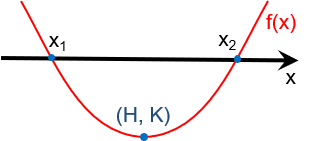Created by Bogna Szyk and Dominik Czernia, PhD candidate
Reviewed by Steven Wooding
Last updated: May 10, 2022

If you need to solve an equation of the form Ax² + Bx + C = 0, this quadratic formula calculator is here to help you. With just a few clicks, you will be able to solve even the most challenging problems. This article describes in detail what is the quadratic formula and what the symbols A, B, and C stand for. It also explains how to solve quadratic equations, which have a negative determinant and no real roots.

## What is the quadratic formula?

The quadratic formula is the solution of a second degree polynomial equation of the following form:

Ax² + Bx + C = 0

If you can rewrite your equation in this form, it means that it can be solved with the quadratic formula. A solution to this equation is also called a root of an equation.

The quadratic formula is as follows:

x = (-B ± √Δ)/2A

where:

• Δ = B² - 4AC

Using this formula, you can find the solutions to any quadratic equation. Note that there are three possible options for obtaining a result:

• The quadratic equation has two unique roots when Δ > 0. Then, the first solution of the quadratic formula is x₁ = (-B + √Δ)/2A, and the second is x₂ = (-B - √Δ)/2A.
• The quadratic equation has only one root when Δ = 0. The solution is equal to x = -B/2A. It is sometimes called a repeated or double root.
• The quadratic equation has no real solutions for Δ < 0.

You can also graph the function y = Ax² + Bx + C. It's shape is a parabola, and the roots of the quadratic equation are the x-intercepts of this function.

💡 We use the quadratic formula in many fields of our life, not only mathematics or physics but also construction. For example, you can plan a smooth transition between two sloped roadways using the vertical curve formula which bases on the quadratic equation.

## Coefficients of a quadratic equation

A, B, and C are the coefficients of the quadratic equation. They are all real numbers, not dependent on x. If A = 0, then the equation is not quadratic, but linear.

If B² < 4AC, then the determinant Δ will be negative. It means that such an equation has no real roots.

## How to use the quadratic formula solver

1. Write down your equation. Let's assume it is 4x² + 3x - 7 = -4 - x.

2. Bring the equation to the form Ax² + Bx + C = 0. In this example, we will do it in the following steps:

4x² + 3x - 7 = -4 - x

4x² + (3+1)x + (-7+4) = 0

4x² + 4x - 3 = 0

3. Calculate the determinant.

Δ = B² - 4AC = 4² - 4*4*(-3) = 16 + 48 = 64.

4. Decide whether the determinant is greater, equal, or lower than 0. In our case, the determinant is greater than 0, which means that this equation has two unique roots.

5. Calculate the two roots using the quadratic formula.

x₁ = (-B + √Δ)/2A = (-4 +√64) / (2*4) = (-4+8) / 8 = 4/8 = 0.5

x₂ = (-B - √Δ)/2A= (-4 -√64) / (2*4) = (-4-8) / 8 = -12/8 = -1.5

6. The roots of your equation are x₁ = 0.5 and x₂ = -1.5.

You can also simply type the values of A, B, and C into our quadratic equation calculator and let it perform all calculations for you.

Make sure you have written down the correct number of digits using our significant figures calculator.

## Solving quadratic equations with a negative determinant

Even though the quadratic formula calculator indicates when the equation has no real roots, it is possible to find the solution of a quadratic equation with a negative determinant. These roots will be complex numbers.

Complex numbers have a real and imaginary part. The imaginary part is always equal to the number i = √(-1) multiplied by a real number.

The quadratic formula remains the same in this case.

x = (-B ± √Δ)/2A

Notice that, as Δ < 0, the square root of the determinant will be an imaginary value. Hence:

Re(x) = -B/2A
Im(x) = ± (√Δ)/2A

## Extra resources

An alternative way of dealing with quadratic equations is factoring trinomials. And it really helps if you're able to quickly recognize perfect square trinomials. The next step is to learn how to graph quadratic inequalities.

If, after learning all about solving quadratic equations, you still want more math? Omni has over to explore. In particular, we recommend you take a look at our cubic equation solver. We also recommend you to check out the . They have a vast collection of resources to learn all about math, with particular attention to the Common Core curriculum.

Bogna Szyk and Dominik Czernia, PhD candidate
Select formula and enter parameters
Formula form
Ax² + Bx + C = 0
A
B
C
Results
Allow negative discriminant
No
Discriminant (Δ)
Standard form: f(x) = Ax² + Bx + C
Vertex form: f(x) = A(x - H)² + K
Factored form: f(x) = A(x - x₁)(x - x₂)People also viewed…

### Black Hole Collision Calculator

The Black Hole Collision Calculator lets you see the effects of a black hole collision, as well as revealing some of the mysteries of black holes, come on in and enjoy!

### Circle measurements

Our circle measurements calculator helps to calculate the radius, diameter, circumference and area of a circle.

### Sleep

The sleep calculator can help you determine when you should go to bed to wake up happy and refreshed.

### Unit circle

Learn about the trigonometric functions with this unit circle calculator.